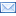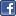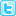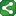## Thursday, August 7, 2008

### Short Code Samples

1) IF-THEN-ELSE vs SELECT:

The SELECT Loop Multiple IF statements in a DATA Step can be replaced with the more efficient SELECT Loop. If you would normally write:

if region='South' then quarter=4;
else if region='North' then quarter=3;
else if region='East' then quarter=2;
else quarter=1;

You can accomplish the same thing with the SELECT Loop in a DATA Step:

select(region);
when('South') quarter=4;
when('North') quarter=3;
when('East') quarter=2;
otherwise quarter=1;
end;

source:www.usc.edu

2) IFC and IFN functions:new IF functions:

IFN(condition, true-numeric-value, false-numeric-value, missing-numeric-value):IFN returns a numeric value. It returns the true, false or missing value depending on whether the condition is true, false or missing.

IFC(condition, true-character-value, false-character-value, missing-character-value):IFC returns a character value. It returns the true, false or missing value depending on whether the condition is true, false or missing.

The IFN function is similar to the IFC function, except that IFN returns a numeric value whereas IFC returns a character value.

IFN evaluates the first argument, then logical-expression. If logical-expression is true (that is, not zero and not missing), then IFN returns the value in the second argument. If logical-expression is a missing value, and you have a fourth argument, then IFN returns the value in the fourth argument.

If logical-expression is false, IFN returns the value in the third argument. The IFN function, an IF/THEN/ELSE construct, or a WHERE statement can produce the same results However, the IFN function is useful in DATA step expressions when it is not convenient or possible to use an IF/THEN/ELSE construct or a WHERE statement.

source: whatsnew_10110.pdf from www.sas.com

Without IFC:

data new;
set old;
if safarmcd='A' then ord=1;
else if safarmcd='B' then ord=2;
run;

with IFC:

data new;
set old;
ord=ifc(safarmcd='A',1,2);
run;

without IFC:

data percent;
length para \$20;
if arm='placebo' then percent=100*count/&a;
if arm='drug' then percent=100*count/&b;
if arm='total' then percent=100*count/&c;

if percent>=99.5 then para=put(count,4.)'('put(percent,5.1)'%)';
else if percent>=9.95 then para=put(count,4.)' ('put(percent,4.1)'%)';
else if percent>. then para=put(count,4.)' ('put(percent,3.1)'%)';
run;

With IFC:

data percent;
length para \$20;
if arm='placebo' then percent=100*count/&a;
if arm='drug' then percent=100*count/&b;
if arm='total' then percent=100*count/&c;

if percent>=99.5 then para=put(count,4.)'('put(percent,5.1)'%)';
para=IFC(percent>=9.95,put(count,4.)' ('put(percent,4.1)'%)',put(count,4.)' ('put(percent,3.1)'%)');
run;

#### 2 comments:

Views said...

Can u plz give in more detail for the last example of ifc.
Itried it but it gives an error "too many arguments for function IFC "
What abt the condition percent > 99.5 ?

sarath said...

since IFN and IFC option is useful for 2 arguements, if u come across a situation where you need to think abt. 3 arguments, you can use IFN and IFC for First 2 arguments and use IF statement for the 3rd or else use IF statements for all the 3 arguments.

*IFC and IFN options are suitable for 2 arguments.....

#### Post a CommentShareThis